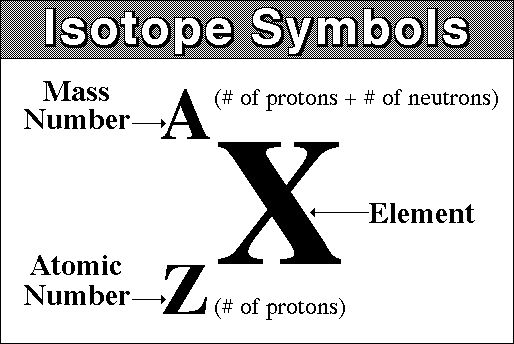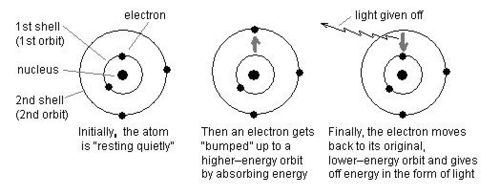# Structure of an Atom

## Inside an Atom

Atoms make up all matter in the universe, they are the building blocks of matter. Atoms, however, are not the smallest particles and even the building blocks have building blocks.Inside the nucleus there are protons and neutrons. Protons have a positive charge (+1) and neutrons have a neutral charge (0). As a result the overall charge of the nucleus is positive.

Electrons have a negative charge (-1) and these are found around the nucleus of the atom in different energy shells.

The key information for subatomic particles are shown in the table below.An atom normally has the same number of protons and electrons. This means that the overall charge of an atom is zero or neutral. This is because the charge from all of the protons combined ‘cancels out’ the overall charge from all of the electrons.

## Size

Atoms are incredibly small! In fact they are 1 x 10-10 or 0.0000000001m.

Atoms are mostly made up of empty space. The radius of a nucleus is around 10,000 times smaller than the radius of an atom.

Small molecules are also the same sort of size. A simple water molecule made up of one oxygen and two hydrogens is 2.75 x 10-10 m.

﻿

## Index Notation

The constituents of atoms are shown in a particular way known as nuclide notation. The general form is given below:As above, the mass number is both protons and neutrons and the atomic number is just protons. Sometimes the atomic number is also called the proton number.

If you need to work out how many neutrons there are in an atom then you need to minus the atomic number from the mass number or:

Number of neutrons = A - Z

Let’s look at an example:This tells us that there are

• Protons: 17
• Neutrons: 35 - 17 = 18
• Electrons: 17 (Remember same as the number of protons)
1. Protons = 2, Electrons = 2, Neutrons = 2
2. Protons = 7, Electrons = 7, Neutrons = 7
3. Protons = 9, Electrons = 9, Neutrons = 8
4. Protons = 11, Electrons = 11, Neutrons = 12
5. Protons = 19, Electrons = 19, Neutrons = 20

## Isotopes

An isotope is an element that has a different number of neutrons but the same number of neutrons. This means that not all elements have the same number of neutrons, while they must have the same number of protons.

All atoms are isotopes.

## Electron Movement

Changes within atoms and the nuclei of atoms can result in EM waves being produced or absorbed over a wide range of frequencies.Gamma rays are produced in the __nucleus __only.

## Ions

In the event where an atom has gained or lost electrons, it is no longer neutrally charged and is called an ion.

• If an isotope has gained electrons it is a negative ion.
• If an isotope has lost electrons it is a positive ion.
What particles are found inside the nucleus?
Your answer should include: protons / neutrons
What happens if an electron emits electromagnetic radiation?
Your answer should include: towards / nucleus
Explanation: It moves towards the nucleus
What is the size of an atom? a) 1 x 10^-10m, b) 1 x 10^-11m, c) 1 x 10^-9m, d) 10 x 10^-10m
a